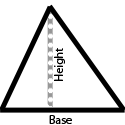## Area of a Triangle

### Area: Learn

Area is the space within a shape. The units of area are the squares of the units of length (that is, if a shape's sides are measured in meters, then the shape's area is measured in square meters).

Triangles have an interesting relationship with rectangles. You can see in the diagram below how a triangle can be made by cutting a rectangle in half along a diagonal.So, finding the area of a triangle uses the same base times height multiplication as a rectangle, but then divide by 2 to find the area of just the triangle. Here is an example: Find the area of a triangle with a base of 4m and a height of 5m.

• Multiply the base by the height: 4*5 = 20
• Divide the result in half: 20÷2 = 10m2

Any side of the triangle can be used as the base, but then the height must be the line which is drawn from the base side to the opposite angle (vertex). This is shown in the diagram below.As a shortcut to keep the numbers small, you can divide either the base or height by two before multiplying. Here is an example using the same triangle as above (4m base and 5m height):

• Instead of dividing by 2 at the end, let's divide the 4 first: 4÷2=2
• Now multiply the base and height: 2*5 = 10m2

### Area: Practice

00:00

What is the area of a triangle whose
base length is cm
and
height length is cm?

Press the Start Button To Begin

You have 0 correct and 0 incorrect.

This is 0 percent correct.

### Play

Game Name Description Best Score
How many correct answers can you get in 60 seconds? 0
Extra time is awarded for each correct answer.
Play longer by getting more correct.
0
How fast can you get 20 more correct answers than wrong answers? 999